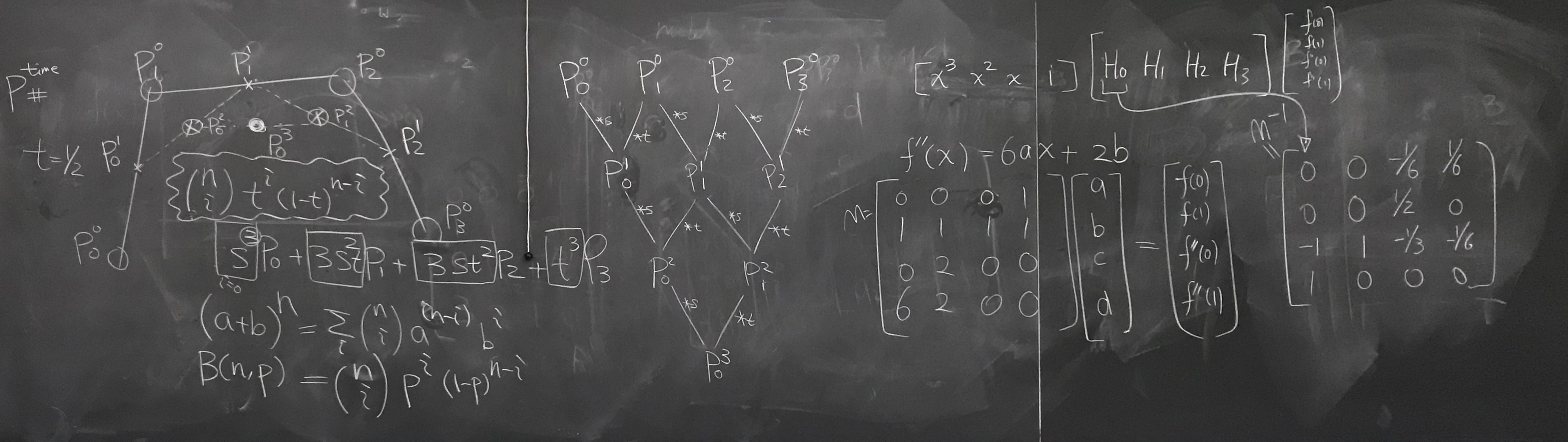Discussion 4

Bullets

• Geometry representations (in discussion 4)
• Explicit
• Point cloud
• Polygon mesh
• Subdivisions
• NURBS
• Implicit
• Level sets
• Algebraic surface
• Distance functions
• etc.
• Topology vs Geometry
• 2D Manifold
• Surface that when cut with a sphere always yields a disk
• Half-edge data structure
• Operations
• Edge flip
• Edge split
• Edge collapse
• Geometry processing
• Ray tracing
• Ray equation
• Plane equation
• Distance from origin to plane?
• Ray-plane intersection
• Ray-triangle intersectioon (moved to discussion 5)
• Möller Trumbone Algorithm
• Ray-sphere intersection (skipped)
• Ray intersection with implicit surfaces (skipped)

Half-edge data structure

🖍 Board work on half-edge data structure

See below for a minimal representation of the half-edge data structure. You may assume the winding are counter-clockwise for the half-edges.

struct Halfedge {
HalfedgeIter &next();
HalfedgeIter &twin();
VertexIter &vertex(); // At its base
EdgeIter &edge();
FaceIter &face();
};

struct Vertex {
HalfedgeIter &halfedge(); // Any half-edge going out from this vertex
Vector3D position;
};

struct Edge {
HalfedgeIter &halfedge(); // Half-edge on either side
};

struct Face {
HalfedgeIter &halfedge(); // Half-edge along any edge
};

Ray tracing

🖍 Board work on ray intersection mathBoard work at the end of section